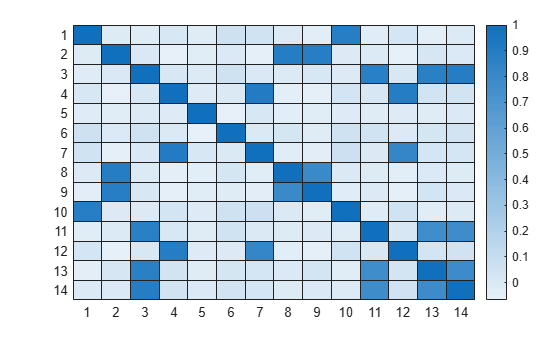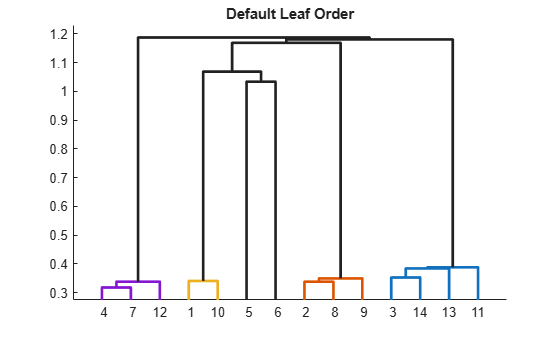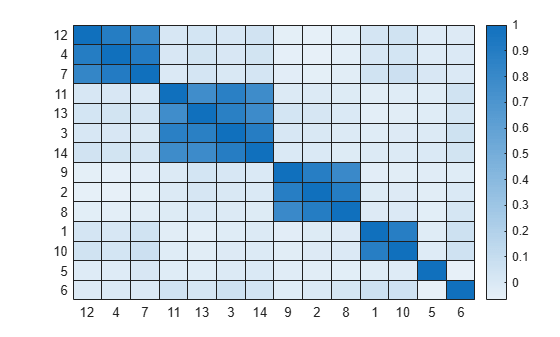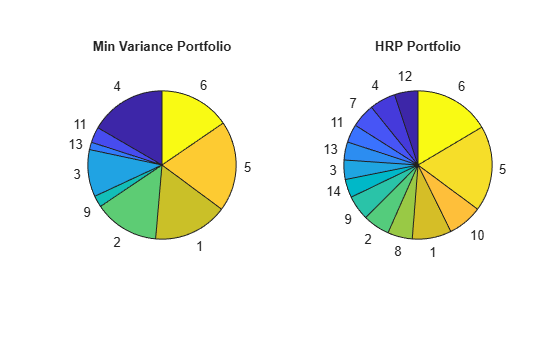# Create Hierarchical Risk Parity Portfolio

This example shows how to compute a hierarchical risk parity (HRP) portfolio. You can use HRP as a technique for portfolio diversification where the assets are divided and weighted according to a hierarchical tree structure. The weights of the assets within a cluster and between clusters can be assigned in many ways. A few ideas of the ways to allocate the weights are:

• Compute an inverse variance portfolio within each cluster. Then, allocate weights to each cluster using a value proportional to the inverse of the variance of the cluster's portfolio.

• Compute a risk parity portfolio within each cluster. Then, use a risk parity allocation strategy to assign each cluster's weights. The risk parity between clusters uses the covariance matrix between the cluster's portfolios. This example focuses on this allocation strategy.

• Use a bisection approach like the one described in Lopez de Prado . For more information, see the example Asset Allocation - Hierarchical Risk Parity.

Begin by loading the data and looking at the correlation between the assets returns.

```% Load data assetRetn = readmatrix("./retns_assets.txt"); [nSample,nAssets] = size(assetRetn); % Compute covariance and correlation matrices Sigma = cov(assetRetn); C = corrcov(Sigma); heatmap(C);```### Hierarchical Clustering

Hierarchical clustering is a common clustering technique in machine learning. In the context of asset allocation, a hierarchical clustering algorithm is applied to find the distance or similarity between each pair of assets and group them into a multilevel binary hierarchical tree.

Begin by defining a measure of likeness or distance between the assets. The more correlated two assets are, the closer they should be.

```% Compute the correlation distance matrix distCorr = ((1-C)/2).^0.5;```

Use the `linkage` function to compute the matrix that encodes the hierarchical tree of the assets in the universe. Them use the `dendrogram` function to visualize the hierarchical structure.

```% Compute the linkage link = linkage(distCorr); figure; h = dendrogram(link, ColorThreshold='default'); set(h, LineWidth=2); title('Default Leaf Order');```The covariance or correlation matrix can be rearranged to be very close to a block diagonal matrix using the information obtained from the hierarchical tree. Each block in the diagonal shows the assets that are closely related.

```% Sort assets for quasi-diagonalization nLeafNodes = size(link,1) + 1; rootNodeId = 2*nLeafNodes - 1; sortedIdx = getLeafNodesInGroup(rootNodeId,link); heatmap(C(sortedIdx,sortedIdx), XData=sortedIdx, YData=sortedIdx);```The plot shows that there are 6 blocks of assets that are closer together. You can divide the assets into 6 clusters using the `cluster` function.

```% Get clusters T = cluster(link, MaxClust=6);```

### Hierarchical Risk Parity Algorithm

Given a clustering of the assets, the HRP algorithm presented in this example follows these steps:

1. Build a risk parity portfolio within each cluster. The `hrpPortfolio` function in Local Functions computes the HRP portfolio by receiving a vector with the cluster assignment and a covariance matrix $\Sigma$. Then, a risk parity portfolio is computed within each cluster by using `riskBudgetingPortfolio`. The `riskBudgetingPortfolio` function receives a reduced covariance matrix that only includes the information of the assets within the cluster and it returns the weights of the assets in the cluster

${\mathit{w}}^{\mathit{j}}=$`riskBudgetingPortfolio(`${\Sigma }^{\mathit{j}}$`)`,

` `where ${\Sigma }^{\mathit{j}}$ is a matrix whose entries include the covariance information only for the assets in the $\mathit{j}$th cluster.

2. Compute each cluster's weight using the covariance between each cluster's portfolio. Now, the `riskBudgetingPortfolio` function receives a matrix ($\Gamma$) that represents the covariance between

the cluster's portfolios (${\mathit{w}}^{\mathit{j}}$).

${\Gamma }_{\mathrm{ij}}={\mathit{W}}^{\mathit{T}}\Sigma \mathit{W}$,

where ${\mathit{W}}_{\mathrm{ij}}={\mathit{w}}_{\mathit{i}}^{\mathit{j}}$ if asset $\mathit{i}$ is in cluster $\mathit{j}$, otherwise ${\mathit{W}}_{\mathrm{ij}}=0$. In other words $\mathit{W}=\left({\mathit{w}}^{1}|...|{\mathit{w}}^{\mathit{K}}\right)$, for $\mathit{K}$ clusters and $\gamma =$`riskBudgetingPortfolio(`$\Gamma$`).`

3. The final asset allocation is given by the cluster's risk parity portfolio (${\mathit{w}}^{\mathit{j}}$) multiplied by each cluster's weight (${\gamma }_{\mathit{j}}$)

$\mathit{w}=\mathit{W}\gamma$.

```% Compute HRP portfolio wHRP = hrpPortfolio(T,Sigma)```
```wHRP = 14×1 0.0854 0.0584 0.0423 0.0567 0.1867 0.1648 0.0521 0.0540 0.0539 0.0755 ⋮ ```

### Compare HRP and Mean-Variance Portfolios

Define a long-only, fully-invested mean-variance `Portfolio` object. Then, compute the associated minimum variance portfolio.

```% Define Portfolio object p = Portfolio(AssetMean=mean(assetRetn), AssetCovar=Sigma); p = setDefaultConstraints(p); % long-only, fully-invested portfolio % Min variance portfolio wMV = estimateFrontierLimits(p,'min');```

Visualize the resulting allocations from these two strategies.

```% Create pie chart labels to improve plot reading labels = 1:nAssets; labels = string(labels); % Sort assets following quasi-diagonalization order labels = labels(sortedIdx); wMV = wMV(sortedIdx); wHRP = wHRP(sortedIdx); % Plot pie charts tiledlayout(1,2); % Min variance portfolio nexttile pie(wMV(wMV>=1e-8),labels(wMV>=1e-8)) title('Min Variance Portfolio',Position=[0,1.5]); % HRP portfolio nexttile pie(wHRP,labels) title('HRP Portfolio',Position=[0,1.5]);```You can see that the HRP portfolio is a much more diversified portfolio as compared to the portfolio obtained using the traditional mean-variance approach. In addition, you can see the following:

• The assets that were not correlated with others, assets 5 and 6, represent a much larger area of the pie. In fact, the sum of the areas of assets that are in the same cluster (for example, assets 1 and 10 or assets 11, 13, 3 and 14) are close to the individual areas of assets 5 and 6. This shows that the weights are divided somewhat evenly between clusters and that each cluster's weight is divided somewhat evenly among the assets within the cluster. This is consistent with the HRP theory.

• The risk parity portfolio of fully correlated assets with the same variance is an equally weighted portfolio. The same happens for assets that are completely uncorrelated. Since all the assets in the universe have a similar variance, and assets between clusters are almost uncorrelated, the weights allocated to each cluster are almost even. And, since the assets within a cluster are meant to be highly correlated, the weights of the assets within a cluster are evenly distributed.

### References

1. Lopez de Prado, M. "Building Diversified Portfolios That Outperform Out of Sample." The Journal of Portfolio Management. 42(4), 59-69, 2016.

### Local Functions

```function pwgt = hrpPortfolio(T,Sigma) % Function that computes a hierarchical risk parity portfolio. The % algorithm first computes a risk parity portfolio for each cluster. Then, % each cluster is assigned a weight based on a risk parity allocation of % the covariance between the cluster's portfolios. % Get the problem information. nAssets = size(Sigma,1); nClusters = max(T); % Compute the risk parity portfolio within each cluster. W = zeros(nAssets,nClusters); for i = 1:nClusters % Identify assets in cluster i and the sub-covariance matrix. idx = T == i; tempSigma = Sigma(idx,idx); % Compute the risk parity portfolio of cluster i. W(idx,i) = riskBudgetingPortfolio(tempSigma); end % Compute the covariance between the risk parity portfolios of each % cluster. covCluster = W'*Sigma*W; % Compute the weights of each cluster. wBetween = riskBudgetingPortfolio(covCluster); % Multiply the weight assigned to each cluster with its portfolio and % assign to the corresponding assets. pwgt = W*wBetween; end function idxInGroup = getLeafNodesInGroup(nodeId, link) % getLeafNodesInGroup finds all leaf nodes for a given node id % in a linkage matrix. nLeaves= size(link, 1)+1; if nodeId > nLeaves tempNodeIds = link(nodeId-nLeaves,1:2); idxInGroup = [getLeafNodesInGroup(tempNodeIds(1), link), ... getLeafNodesInGroup(tempNodeIds(2), link)]; else idxInGroup = nodeId; end end```